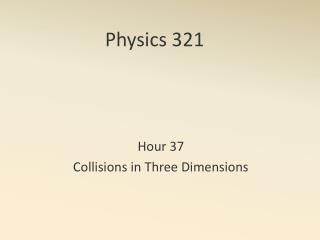DownloadDownload PresentationPhysics 321

# Physics 321

Download Presentation## Physics 321

- - - - - - - - - - - - - - - - - - - - - - - - - - - E N D - - - - - - - - - - - - - - - - - - - - - - - - - - -
##### Presentation Transcript

1. Physics 321 Hour 37 Collisions in Three Dimensions

2. Assume we know the masses, the initial momentum of each body (they’re the same), and the scattering angle. Collision in the CM Frame Θ Θ

3. Assume we know any energy loss as well. Collision in the CM Frame • We can find the kinetic energies is we know the momenta and the masses.

4. First, we find P. Collision in the CM Frame • Then we find T1 and T2. • Now we know everything!

5. If Eex=0, Elastic Scattering • All the scattering can do is change the directions of the particles.

6. The cm frame is really easy… • Theorists (almost) always work in the cm frame. • Experimentalists usually convert cross sections to the cm as well. • But, experiments are not usually conducted in the cm frame! The CM Frame

7. Now let’s assume we have the CM solution and we want to get back to the lab frame. • We know CM to Lab

8. So we can find the lab quantities CM to Lab

9. CM to Lab

10. But usually we don’t know Θa priori. CM to Lab This is a quadratic equation we can solve for p1. Then we can find Θand everything else.

11. CollisionKinematics.nb Example

12. Physics 321 Hour 38 Scattering Cross Sections

13. In the cm frame, two particles approach each other and scatter. Given the interaction, what is the distribution of scattered particles? The Scattering Problem Θ Θ

14. Given the distribution of scattered particles, what is the interaction? • This is a very hard but very interesting problem. The Inverse Scattering Problem Θ Θ

15. The impact need not be head-on. – The distance between the trajectories is the impact parameter, b. The Scattering Problem Θ b Θ

16. The first thing we need to do is find the relationship between θand b for a given type of interaction. Relating θ to b Θ b Θ

17. Hard Sphere Scattering – b(θ)

18. First we note that the total angular momentum is Coulomb Scattering – b(θ)

19. Next we use a result from the central force problem. Coulomb Scattering – b(θ)

20. For hyperbolic orbits Coulomb Scattering – b(θ) φ

21. Using Coulomb Scattering – b(θ) φ

22. Coulomb Scattering – b(θ)

23. Consider a small beam striking a large target. The detector has solid angle ΔΩand detects all particles scattered. Differential Cross Section - Definition detector θ beam target

24. Consider a large beam striking a small target. A detector has solid angle ΔΩ. Differential Cross Section - Definition detector beam θ target

25. We usually take 1 target particle… Differential Cross Section - Theoretical detector beam θ target

26. Differential Cross Section - Theoretical Find the total number of beam particles per second between This is the number in a ring of radius . This is:

27. Differential Cross Section - Theoretical 2) Find the total number of scattered particles between This is:

28. 3) Think of the detector as subtending angles and . The solid angle is defined by: or more generally (If the area of the detector is small, this can be expressed in terms of the detector’s area: .) Differential Cross Section - Theoretical

29. 3) Think of the detector as subtending angles and . The solid angle is defined by: (If the area of the detector is small, this can be expressed in terms of the detector’s area: .) This is: Differential Cross Section - Theoretical

30. 4) The number of particles per second scattering into is: The number of those hitting the detector is Differential Cross Section - Theoretical

31. The number of particles detected per second is Differential Cross Section - Theoretical Or since we want a positive value, we usually write

32. The total cross section is the differential cross section integrated over all angles. Total Cross Section

33. Hard Sphere Scattering (cm)

34. Hard Sphere Scattering (cm)

35. Rutherford Scattering (cm)

36. The only thing that changes in lab vs cm is . CM to Lab Conversion